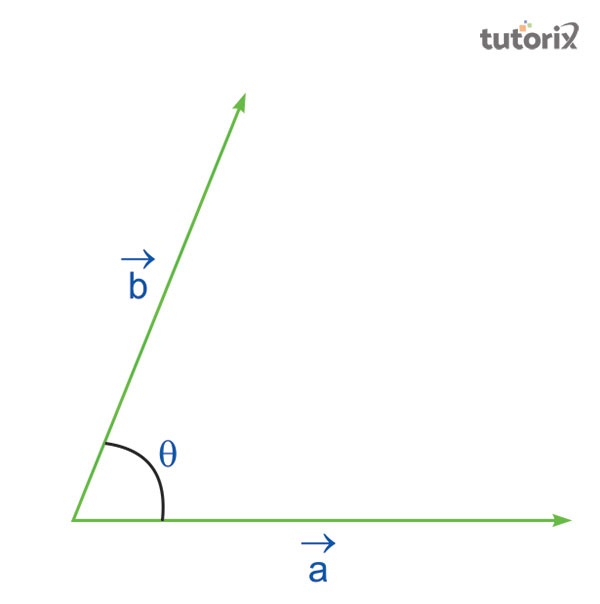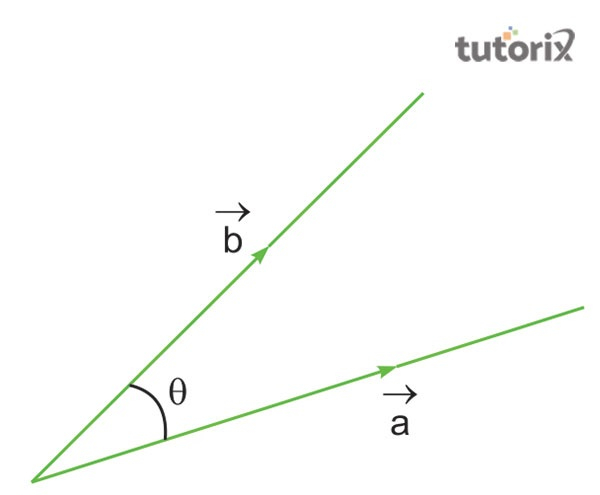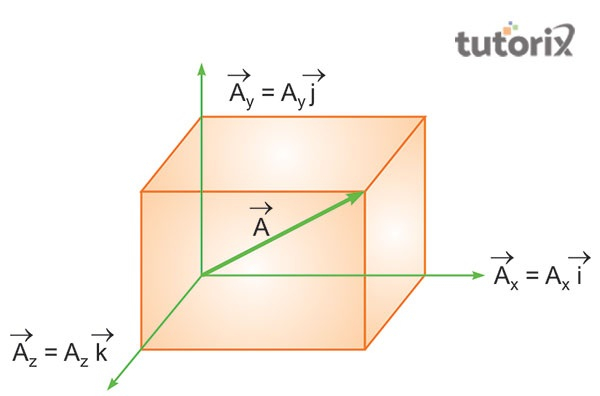# Scalar Product

## Introduction

The scalar products are essential in order to understand the motion of vector quantities in real-time. These products can be subtracted or added at the same time with a proper algebraic formula for understanding the scalar quantity of different objects. These types of products are commonly denoted as the inner products or dot products based on their characteristics to outline scalar multiplication through dots.

## Definition of Scalar Product

The scalar products is defined as the products of magnitude that consists of two vectors cosine angle between different vectors. Scalar products mostly involve the sum of the products for the corresponding entries along with two sequences of numbers. These products have various applications in terms of mechanics, engineering and geometry (Cavaglia et al. 2019).

In simple words, the scalar products can be defined as the products of magnitude that has two different vectors and one angle between them.Figure 1: Scalar Products example 1

For example, if $\mathrm{\vec{a}}$ and $\mathrm{\vec{b}}$ are two non-zero vectors that have a certain magnitude of |a| and |b| with an angle of θ, then the algebraic operation for the solar product will be "$\mathrm{\vec{a}.\vec{b} \:= \:|a| |b| \:cos\: θ}$. Here, the expression denotes 0 ≤ θ ≤ according to the rule of scalar products. Here, either a or b is equal to 0, especially when θ is not defined within the equation. Under this circumstance, both a and b in this scalar product appear as equal to 0 (Sciencedirect, 2022).

So, if the value of vector a and vector b equals 0 and then the value of vector a.b also stands at the value of 0.Figure 2: Scalar Products example 2

In the case of the second example, it can be seen that if two vectors, “$\mathrm{\vec{a}}$ and “$\mathrm{\vec{b}}$ are drawn to the θ of the scalar product, the scalar product can appear as $\mathrm{\vec{a}.\vec{b} \:= \:|a| |b| \:cos\: θ}$. Here, the |a| is the magnitude of vector a, the |b| signifies the modulus of vector b and the θ represents the angle between vector a and vector b (Mathcentre, 2022).

Due to the symbolic representation of the scalar products as a dot, it is further denoted as dot products while using them in real-time. Components like area, volume, work, energy, pressure, mass, density, time and distance are prime examples of scalar products.

## Matrix representation of Scalar Products

The representation of scalar products on a matrix can be done using two different patterns, the column matrix and the row matrix. The unit vectors of scalar products can be ordinarily spatial with different vectors in the column matrices. Here, the matrix involves components like x, y and z to transpose the vectors in the row matrices (Pei & Terras, 2021). For example, if A and B are two different vectors present in the matrix of scalar products, then both the matrix will collaboratively deliver only one number at a time as a result of the addition and subtraction of two vectors within the scalar products.

$$\mathrm{(A_X | A_Y | A_Z)\begin{pmatrix}B_X \B_Y \B_Z \\end{pmatrix} \:=\: A_X B_X+A_Y B_Y+A_Z B_Z \:=\: \vec{A}. \: \vec{B}}$$

The single number that is commonly extracted from the scalar matrix, generally involves the sum of the products, especially while corresponding to the spatial components of more than one vector at a time. Hence, the matrix representation of scalar products is represented with an illustrious process that is used in the multiplication of matrix within a certain element. Here, the sum of the products is presented in a column and a row format with a certain given number within the scalar products in real-time.

## Characteristics of Scalar products

Scalar products have a few distinctive characteristics that make them different from the vector products in real-time. The primary characteristic of the scalar products, are commutatively and distributivity.

Here the calculation of the scalar properties is done in a different order starting from vector b to vector a, exactly opposite from the general scalar products. Apart from that, this type of product generally follows the distributive law that can be implied in three consecutive vectors, a, b and c.Figure 3: Scalar products characteristics

The scalar products can be defined based on their magnitude only along with the algebraic solutions related to the addition and subtraction of vectors in real-time. Another characteristic of scalar products is the manual perpendicular standing of the vectors at a time, especially when the value of two vectors stands on 0 only (Thefactfactor, 2022). Lastly, the square of the magnitudes of the vector stands equally with the self-product of a vector according to the commutative law of scalar products.

## Conclusion

Scalar products are commutative products along with the incorporation of equal-length number sequences. These products generally return only one number after taking two vectors at a time. Most importantly, the scalar products can be subtracted and added from one another on the basis of algebraic equations in terms of mathematical representation. Moreover, the scalar products are the communicative products that have direct involvement in the alteration of vector components in real-time.

## FAQs

Q1. What is the algebraic formula of Scalar products?

Ans. The algebraic formula of scalar products is |a| |b| cos θ. The formula is dependent on two vectors, vector a and vector b and follows the magnitude of |a| and |b|.

Q2. Which law do the scalar products follow?

Ans. The scalar products generally follow two distinctive rules, the commutative law and the distributive law. The distribution of these products follows the approach related to addition whereas the commutative law follows the commutative approach.

Q3. What are some examples of scalar products in real-time?

Ans. Scalar products have real-time usages starting from deciding routes and searching the routes in a particular place. Apart from that, there is major usage of these products in the fields of calculating the Pythagoras theorem in real life.